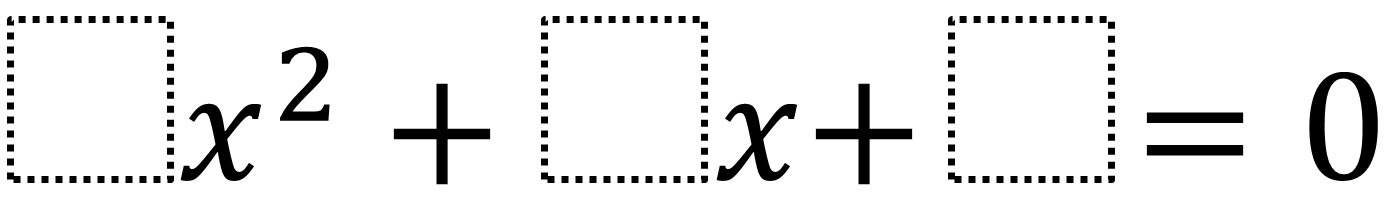Home > High School: Algebra > Factoring Quadratics – Fraction Solution

# Factoring Quadratics – Fraction Solution

Directions: Using the digits 0 to 9 at most one time each, fill in the boxes to so that at least one of the solutions is a fraction.### Hint

How could you find the equation if you already knew the solutions?

### Answer

There are many answers including 4x^2 + 5x + 1 which has solutions of -1/4 and -1.

Source: Daniel Luevanos

## Writing Equivalent Polynomial Expressions

Directions: Use the digits 1-9, at most one time each, to create a true statement. …